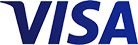Grid List
\$261.05 inc GST (Each)
Increase value Decrease value
\$344.40 inc GST (Each)
Increase value Decrease value
\$16.18 inc GST (Pair)
Increase value Decrease value
\$39.40 inc GST (Each)
Increase value Decrease value
\$36.28 inc GST (Each)
Increase value Decrease value
\$22.30 inc GST (Pair)
Increase value Decrease value
\$3.41 inc GST (Each)
Increase value Decrease value
\$31.64 inc GST (Each)
Increase value Decrease value
\$41.64 inc GST (Each)
Increase value Decrease value
\$53.46 inc GST (Each)
Increase value Decrease value
\$31.57 inc GST (Each)
Increase value Decrease value
\$37.02 inc GST (Each)
Increase value Decrease value